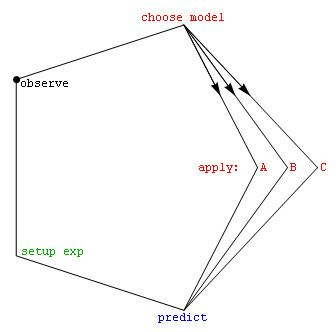# Model selection

Updated on
Covid-19Model selection is the task of selecting a statistical model from a set of candidate models, given data. In the simplest cases, a pre-existing set of data is considered. However, the task can also involve the design of experiments such that the data collected is well-suited to the problem of model selection. Given candidate models of similar predictive or explanatory power, the simplest model is most likely to be the best choice.

## Contents

Konishi & Kitagawa (2008, p. 75) state, "The majority of the problems in statistical inference can be considered to be problems related to statistical modeling". Relatedly, Sir David Cox (2006, p. 197) has said, "How [the] translation from subject-matter problem to statistical model is done is often the most critical part of an analysis".

## Introduction

In its most basic forms, model selection is one of the fundamental tasks of scientific inquiry. Determining the principle that explains a series of observations is often linked directly to a mathematical model predicting those observations. For example, when Galileo performed his inclined plane experiments, he demonstrated that the motion of the balls fitted the parabola predicted by his model.

Of the countless number of possible mechanisms and processes that could have produced the data, how can one even begin to choose the best model? The mathematical approach commonly taken decides among a set of candidate models; this set must be chosen by the researcher. Often simple models such as polynomials are used, at least initially. Burnham & Anderson (2002) emphasize throughout their book the importance of choosing models based on sound scientific principles, such as understanding of the phenomenological processes or mechanisms (e.g., chemical reactions) underlying the data.

Once the set of candidate models has been chosen, the statistical analysis allows us to select the best of these models. What is meant by best is controversial. A good model selection technique will balance goodness of fit with simplicity. More complex models will be better able to adapt their shape to fit the data (for example, a fifth-order polynomial can exactly fit six points), but the additional parameters may not represent anything useful. (Perhaps those six points are really just randomly distributed about a straight line.) Goodness of fit is generally determined using a likelihood ratio approach, or an approximation of this, leading to a chi-squared test. The complexity is generally measured by counting the number of parameters in the model.

Model selection techniques can be considered as estimators of some physical quantity, such as the probability of the model producing the given data. The bias and variance are both important measures of the quality of this estimator; efficiency is also often considered.

A standard example of model selection is that of curve fitting, where, given a set of points and other background knowledge (e.g. points are a result of i.i.d. samples), we must select a curve that describes the function that generated the points.

## Methods for choosing the set of candidate models

• Exploratory data analysis
• Scientific method
• ## Criteria for model selection

• Akaike information criterion
• Bayes factor
• Bayesian information criterion
• Cross-validation
• Deviance information criterion
• False discovery rate
• Focused information criterion
• Likelihood-ratio test
• Mallows's Cp
• Minimum description length (Algorithmic information theory)
• Minimum message length (Algorithmic information theory)
• Structural Risk Minimization
• Stepwise regression
• The most commonly used criteria are (i) the Akaike information criterion and (ii) the Bayes factor and/or the Bayesian information criterion (which to some extent approximates the Bayes factor).

## References

Similar Topics
The Stars Fell on Henrietta
Julia Bray
Bruno Renan
Topics